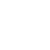Classes (extension) |

# NL : UGen : AbstractFunction : ObjectExtension

Non Linear Filter Equation
Source: SLUGens.sc

//SLUGens released under the GNU GPL as extensions for SuperCollider 3, by Nick Collins, http://composerprogrammer.com/index.html

## Description

Represents the arbitrary non-linear filter difference equation in the time domain:

y(n) = b00x(n)^b01 + b10x(n-1)^b11 + ... + b(Nb0)x(n-Nb0)^Nb1 + a00y(n-1)^a01 + ... + a(Na0)y(n-Na0)^Na1

Though no cross-terms combining powers of x and y are allowed.

Stability is definitely not guaranteed; most equations will quickly blow-up. See the guard arguments below. It is recommended that you stick to positive exponents for signals which are within -1 to 1, else explosion of values is inevitable.

(0.1)**(-1.26) //negative exponents cause blowup for smaller signals abs(sig) < 1.0

(1.1)**(2.26) //positive exponents cause blowup for larger signals abs(sig) > 1.0

You need to pass in the parameters via two buffers, of arbitrary size.

## Class Methods

### NL.ar(input, bufnuma: 0, bufnumb: 1, guard1: 1000.0, guard2: 100.0, mul: 1.0, add: 0.0)

#### Arguments:

 input What do you want to filter? bufnuma Feedback filter coefficients, from previous outputs, in triples of (index,coefficient, exponent) from lowest to highest index bufnumb Feedforward filter coefficients, from previous inputs, in pairs of (index,coefficient, exponent) from lowest to highest index guard1 Watch out for blow-up and reset if necessary; this is the value of the maximum absolute output allowed. guard2 Watch out for blow-up and reset if necessary; this is the value of the maximum absolute change of output allowed. On discovering blow-up, filter output is set back to zero for the last Na stored outputs, so that feedback cannot occur. This UGen can be expensive to run because of the power operations that have to be carried out to calculate each new sample. You can only change the filter equations on the fly where you change multiplier coefficients and exponents; indices are set at initialisation however.